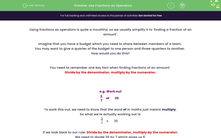# Use Fractions as Operators

In this worksheet, students will find fractions of amounts by applying the rule: 'Divide by the denominator, multiply by the numerator'.Key stage:  KS 4

Year:  GCSE

GCSE Subjects:   Maths

GCSE Boards:   Pearson Edexcel, OCR, Eduqas, AQA,

Curriculum topic:   Number, Fractions, Decimals and Percentages

Curriculum subtopic:   Structure and Calculation Fractions Percentages Fractions, Decimals and Percentages

Difficulty level:#### Worksheet Overview

Using fractions as operators is quite a mouthful, so we usually simplify it to 'finding a fraction of an amount'.

Imagine that you have a budget which you need to share between members of a team.

You may want to give a quarter of the budget to one person and three-quarters to another.

How would you do this?

You need to remember one key fact when finding fractions of an amount:

Divide by the denominator, multiply by the numerator.

e.g. Work out

 2 7
of 35

To work this out, we need to know that the word of in maths just means multiply.

So what we're actually working out is:

 2 7
x 35

If we look back to our rule: Divide by the denominator, multiply by the numerator.

We need to divide 35 by 7 which gives us 5.

We then must multiply 5 by 2 to get 10.

So two-sevenths of 35 is 10.

Job done!

In this activity, you will find fractions of amounts by applying the rule:

Divide by the denominator, multiply by the numerator.

### What is EdPlace?

We're your National Curriculum aligned online education content provider helping each child succeed in English, maths and science from year 1 to GCSE. With an EdPlace account you’ll be able to track and measure progress, helping each child achieve their best. We build confidence and attainment by personalising each child’s learning at a level that suits them.

Get started